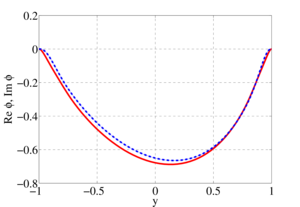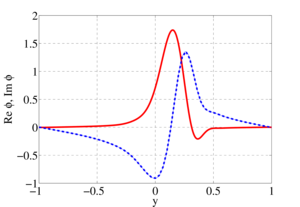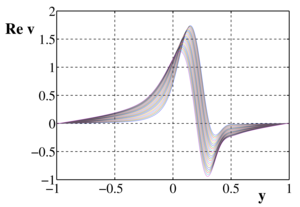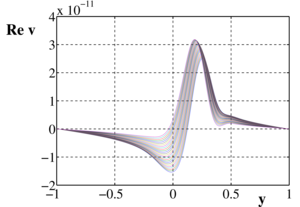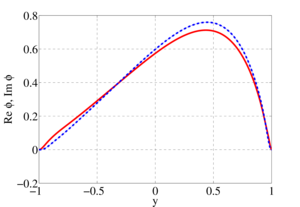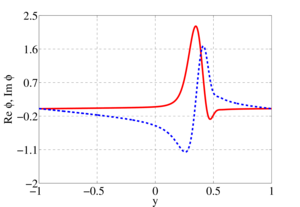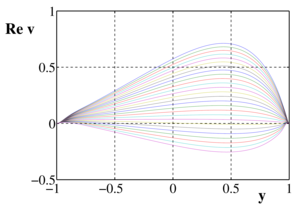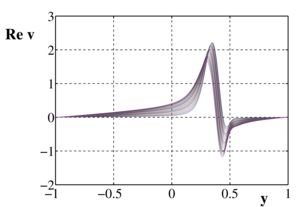ISSN 2658–5782
DOI 10.21662
Electronic Scientific Journal© Институт механики
им. Р.Р. Мавлютова
УФИЦ РАНNizamova A.D., Kireev V.N., Urmancheev S.F. Research of eigenfuctions perturbation of the transverse component velocity thermoviscous liquids flow. Multiphase Systems. 14 (2019) 2. 132–137 (in Russian).
2019. Vol. 14. Issue 2, Pp. 132–137
URL: http://mfs.uimech.org/mfs2019.2.018,en
DOI: 10.21662/mfs2019.2.018
Research of eigenfuctions perturbation of the transverse component velocity thermoviscous liquids flow
Nizamova A.D., Kireev V.N.∗∗, Urmancheev S.F.
Mavlyutov Institute of Mechanics UFRC RAS, Ufa, Russia
∗∗Bashkir State University, Ufa, Russia

### Abstract

The viscous model fluid flow in a plane channel with a linear temperature profile is considered. The problem of the thermoviscous fluid flow stability is solved on the basis of the previously obtained generalized Orr–Sommerfeld equation by the spectral method of decomposition into Chebyshev polynomials. We study the effect of taking into account the linear and exponential dependences of the viscosity of a liquid on temperature on the eigenfunctions of the hydrodynamic stability equation and on perturbations of the transverse velocity of an incompressible fluid in a plane channel when various wall temperatures are specified. Eigenfunctions are found numerically for two eigenvalues of the linear and exponential dependence of viscosity on temperature. Presented pictures of their own functions. The eigenfunctions demonstrate the behavior of the transverse velocity perturbations, their possible growth or attenuation over time. For the given eigenfunctions, perturbations of the transverse flow velocity of a thermoviscous fluid are obtained. It is shown that taking the temperature dependence of viscosity into account affects the eigenfunctions of the equations of hydrodynamic stability and perturbations of the transverse flow velocity. Perturbations of the transverse velocity significantly affect the hydrodynamic instability of the fluid flow. The results show that when considering the unstable eigenvalue over time, the velocity perturbations begin to grow, which leads to turbulence of the flow. The maximum values of the eigenfunctions and perturbations of the transverse velocities are shifted to the hot wall. It is seen that for an unstable eigenvalue, the perturbations of the transverse flow velocity increase over time, and for a stable one, they decay.

Keywords

thermoviscous liquid,
eigenfunctions,
perturbations of cross section velocity,
hydrodynamics instability

## Article outline

The purpose of this work is to research the hydrodynamic stability of thermoviscous liquids flow with monotonic temperature dependences of viscosity and the search for parameters characterizing the flow regimes.

Solution methods. The problem of thermoviscous fluid stability is solved on the basis of the generalized Orr-Sommerfeld equation obtained earlier by the spectral decomposition method in Chebyshev polynomials.

The study found:

1. The thermoviscous fluids flow stability with a linear and exponential dependence of viscosity on temperature is investigated.
2. It is shown that the result of solving the problem of the stability of the flow of thermoviscous liquids is influenced by the selected temperature dependence of the fluid viscosity.
3. The dependence of the eigenfunctions on the values of the thermal viscosity parameter is established.

At present, a sufficient reserve has been accumulated in the study of the stability of flows of liquids in flat channels, however, in studying this problem, the effect of the temperature factor on the change of flow regime is often neglected. Viscous fluid flows occur in a number of industries in the operation of various technical installations and devices, and in the implementation of a number of technological processes. In such cases, the problem of identifying the characteristics of such a flow under various conditions is important. From the point of view of energy efficiency, the laminar regime is important, on the other hand, when considering the efficiency of heat and mass transfer, it is turbulent. Fluid viscosity is an important parameter that determines the flow patterns.

The flow of a viscous model fluid in a plane-parallel channel with a non-uniform temperature field is considered. The influence of taking into account the linear and exponential dependences of the fluid viscosity on temperature on the spectral characteristics of the hydrodynamic stability equation for an incompressible fluid in a flat channel at different wall temperatures is investigated. Analytically obtained profiles of the flow rate of a thermoviscous fluid. At small values of the viscosity change parameter, the velocity profile is similar to the Poiseuille profile, and as it increases, the maximum value of the velocity profile tends towards the heated channel wall. This phenomenon is explained by the fact that the viscosity of the fluid near the hot wall is less than that of the fluid near the cold wall, and therefore the velocity in the region of the heated wall will be greater. The problem of the stability of a thermal viscous fluid is solved on the basis of the generalized Orr-Sommerfeld equation obtained earlier by the spectral decomposition method in Chebyshev polynomials. The spectral pictures of the eigenvalues of the generalized Orr-Sommerfeld equation are constructed. It is shown that the structure of the spectra largely depends on the properties of the liquid, which are determined by the viscosity functional dependence index. It was established that at small values of the thermal viscosity parameter, the spectrum is comparable to the spectrum for isothermal fluid flow, however, as it increases, the number of eigenvalues and their density increase, that is, there are more points at which the problem has a nontrivial solution. The stability of the flow of a thermally viscous fluid depends on the presence of an eigenvalue with a positive imaginary part among the entire set of eigenvalues found with fixed Reynolds number and wavenumber parameters. It is shown that for fixed values of the Reynolds number and wave number with increasing thermal viscosity parameter, the flow can become unstable. The spectral characteristics determine the structure of the eigenfunctions and the critical parameters of the flow of a thermally viscous fluid. In this case, the eigenfunctions demonstrate the behavior of transverse-velocity perturbations, their possible growth or decay over time.

Findings. It has been established that taking into account the dependence of viscosity on temperature rather strongly influences conclusions regarding hydrodynamic stability, which is certainly important when analyzing flow regimes in heat exchangers. With the same values of Reynolds numbers and wave numbers describing stable flow regimes, an increase in the thermal viscosity parameter can lead to unstable modes. It should be noted that in this case a qualitative change occurs in the structure of the eigenfunctions. The spectral characteristics of the flow are an important part in analyzing the flow regimes of liquids.

## References

1. Orszag S.A. Accurate solution of the Orr–Sommerfeld equation // J. of Fluid Mech. 50. 1971. V. 50, No 4 Pp. 689–703.
DOI: 10.1017/S0022112071002842
2. Gol’dshtik M.A., Shtern V.N. [Hydrodynamic stability and turbulence] Novosibirsk: Nauka. 1977. 421 p. (in Russian).
3. Potter M.C., Graber E.J. Stability of plane Poiseuille flow with heat transfer // Nasa technical note. Nasa TN D-6027. 1970. 12 p.
https://ntrs.nasa.gov/archive/nasa/casi.ntrs.nasa.gov/19700031354.pdf
4. Skorohodov S.L. Numerical analysis of the spectrum of the Orr–Sommerfeld problem // Computational mathematics and mathematical physics. 2007. Vol. 47. Issue 10. Pp. 1603–1621.
DOI: 10.1134/S096554250710003X
5. Urmancheev S.F., Kireev V.N. Steady flow of a fluid with an anomalous temperature dependence of viscosity // Doklady Physics. 2004. V. 49, No 5. Pp. 328–331.
DOI: 10.1134/1.1763627
6. Nizamova A.D.[Influence of the temperature dependence of viscosity on the stability of plane-parallel fluid flow] Trudy Instituta mexaniki im. R.R. Mavlutov URC RAS. 2014. No 10. P. 90–94 (in Russian).
DOI: 10.21662/uim2014.1.017
7. Nizamova A.D., Kiree V.N., Urmancheev S.F. [Influence of temperature dependence of viscosity on fluid flow stability] Izvestiya URC RAS. 2014. No 4. Pp. 12–16 (in Russian).
https://elibrary.ru/item.asp?id=24326737
8. Kireev V.N., Nizamova A.D., Urmancheev S.F. [Some features of the hydrodynamic instability of the flow of a thermally viscous fluid in a flat channel] Prikladnaya mexanika i matematika. 2019. Vol. 83, No 3. Pp. 454–459 (in Russian).
DOI: 10.1134/S003282351903007X
9. Nizamova A.D., Kiree V.N., Urmancheev S.F. [Influence of viscosity temperature dependence on the spectral characteristics of the thermoviscous liquids flow stability equation] Multiphyse systems. 2019. Vol. 14, No 1. Pp. 52–58. (in Russian).
DOI: 10.21662/mfs2019.1.007